# Class 8 Maths NCERT Solutions for Chapter – 11 Mensuration Ex – 11.2

## Mensuration

Question 1.
The shape of the top surface of a table is trapezium. Find its area if its parallel sides are 1 m and 1.2 m and perpendicular distance between them is 0.8 m.

Solution:

Area of top surface of a table
= Area of the trapezium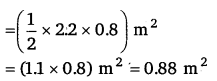Question 2.
The area of a trapezium is 34 cm2 and the length of one of the parallel sides is 10 cm and its height is 4 cm. Find the length of the other parallel side.

Solution:
Let the required side be x cm.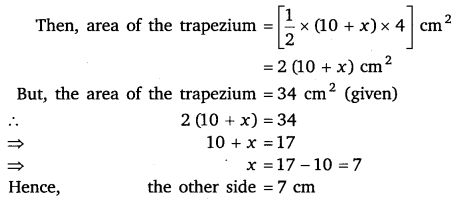Question 3.
Length of the fence of a trapezium A shaped field ABCD is 120 m. IF BC = 48 m, CD = 17 m and AD = 40 m, find the area of this field. Side AB is perpendicular to the parallel sides AD B and BC.

Solution:

Let ABCD be the given trapezium in which BC = 48 m, CD = 17 m and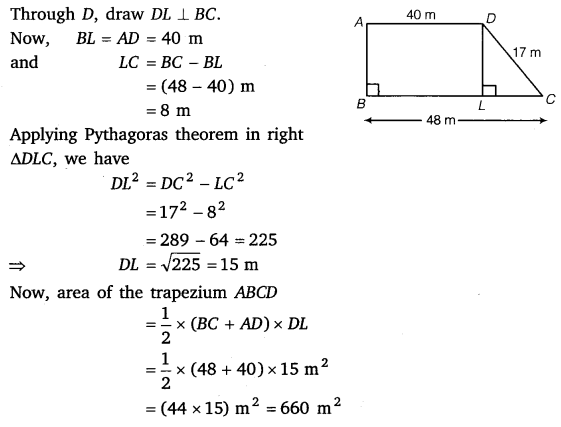Question 4.
The diagonal of a quadrilateral shaped field is 24 m and the perpendiculars dropped on it from the remaining opposite vertices are 8 m and 13 m. Find the area of the field.

Solution:

Let ABCD be the given quadrilateral in which BE ⊥ AC and DF ⊥ AC.
It is given that
AC =24m, BE = 8m and DF = 13 m.
= area of ∆ABC + area of ∆ACDQuestion 5.
The diagonals of a rhombus are 7.5 cm and 12 cm. Find its area.

Solution:
Area of a rhombus =$\frac { 1 }{ 2 }$ x (product of diagonals)$=\left( \frac { 1 }{ 2 } \times 7.5\times 12 \right) { cm }^{ 2 }=45{ cm }^{ 2 }$

Question 6.
Find the area of a rhombus whose side is 6 cm and whose altitude is 4 cm. If one of its diagonals is 8 cm long, find the length of the other diagonal.

Solution:
Let ABCD be a rhombus of side 6 cm and whose altitude DF = 4 cm. Also, one of its diagonals, BD =8 cm.
Area of the rhombus ABCD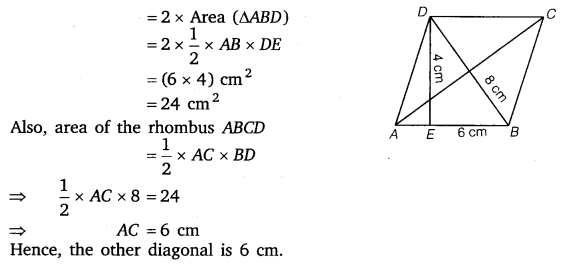Question 7.
The floor of a building consists of 3000 tiles which are rhombus shaped and each of its diagonals are 45 cm and 30 cm in length. Find the total cost of polishing the floor, if the cost per m2 is ₹ 4.

Solution:
Area of the floor = 3000 x Area of one tile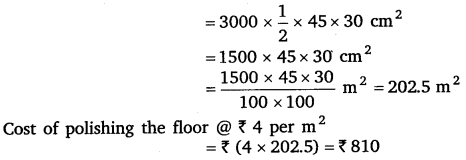Question 8.
Mohan wants to buy a trapezium shaped field. Its side along the. river is parallel to and twice the side along the road. If the area of this field is 10500 m2 and the perpendicular distance between the two parallel sides is 100m, find the length of the side along the river.

Solution:

Let the parallel sides of the trapezium shaped field berm and 2x m. Then, its area∴ The length of the side along the river is 2 x 70, i.e., 140 metres.

Question 9.
Top surface of a raised platform is in the shape of a regular octagon as shown in the figure. Find the area of the octagonal surface.

Solution:
Area of the octagonal surface ABCDEFGH = Area (trap. ABCH) + Area (rect. HCDG) +Area (trap. GDEF)Question 10.
There is a pentagonal shaped park as shown in the figure. For finding its area Jyoti and Kavita divided it in two different ways.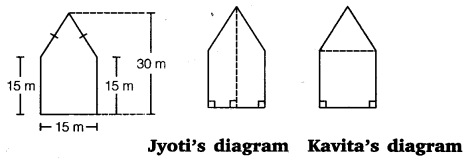Find the area of this park using both ways. Can you suggest some other way of finding its area?

Solution:
Taking Jyoti’s diagram :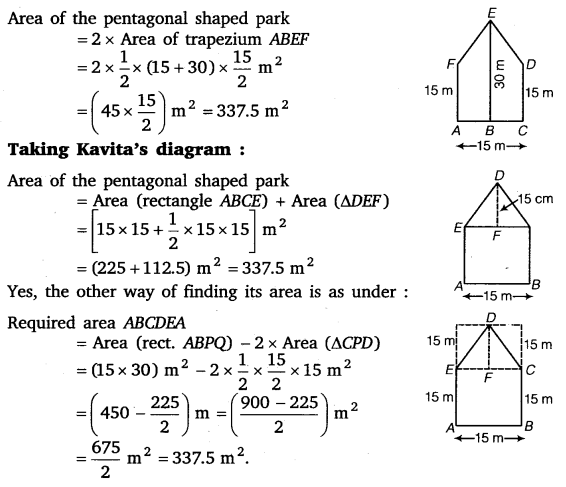Question 11.
Diagram of the adjacent picture frame has outer dimensions = 24 cm x 28 cm and inner dimensions 16 cm x 20 cm. Find the area of each section of the frame, if the width of each section is same.

Solution: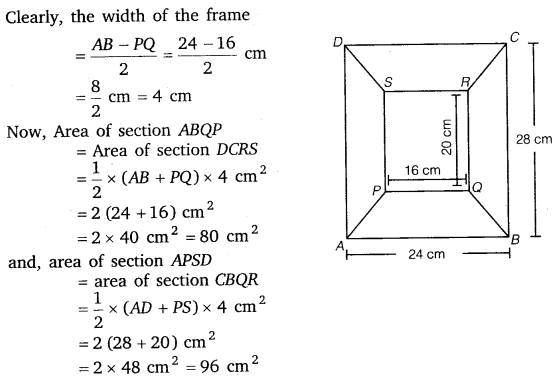### Take Your IIT JEE Coaching to Next Level with Examtube

• Mentoring & Teaching by IITians
• Regular Testing & Analysis
• Preparation for Various Engineering Entrance Exams
• Support for School/Board Exams